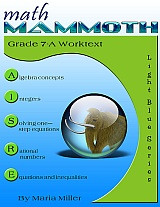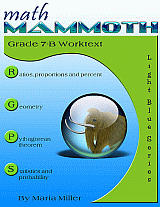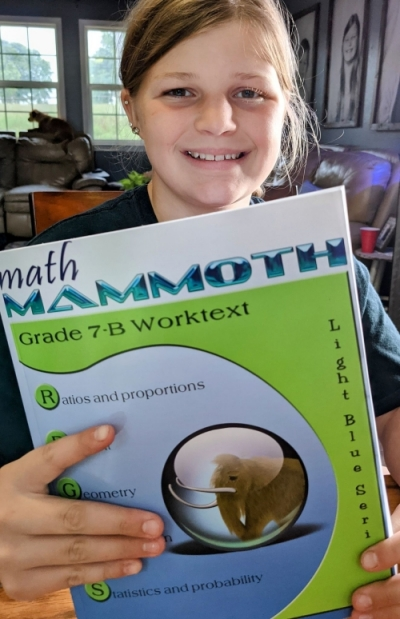Thanksgiving SALE! Get Math Mammoth and Make It Real Learning digital downloads at 30% off — right here at the MathMammoth.com website. Use coupon code THANKS30 at checkout. Offer valid through December 2, 2023.

^
You are here: HomeLight Blue Series → Grade 7

# Math Mammoth Grade 7 complete curriculum

Math Mammoth Grade 7 Complete Curriculum is a complete pre-algebra program for 7th grade. It fully prepares students for an algebra 1 program.227 pages
201 lesson pages292 pages
258 lesson pages

## Prices & ordering

#### Digital version

(the two worktexts, answer keys, tests, cumulative reviews, a worksheet maker, Soft-Pak)

Part 7-A only:

(Everything for the first half of 7th grade math; includes Soft-Pak)

Part 7-B only:

(Everything for the second half of 7th grade math; includes Soft-Pak)

#### CD

The CD contains the same files as the digital download: the two worktexts, answer keys, tests, cumulative reviews, a worksheet maker, Soft-Pak.

#### Printed copies

Rainbow Resource Center sells perfect-bound texts either with full color or with grayscale interior pages:

Lulu sells spiral (coil) bound versions of the student books and of the tests & reviews book.

Supportive materials

This product is meant to go with the printed student books, and includes digital versions of the answer keys, tests, cumulative revisions, worksheet maker and software Soft-Pak (USD \$9.60).

Do NOT purchase if you're getting the answer keys and tests & cumulative revisions as printed books, or if you're getting the digital version.

#### Lesson plan

You can purchase a lesson plan for Math Mammoth Grade 7 to use it in Homeschool Planet online planner. Learn more.

#### Skills Review WorkbookThis workbook is supplemental, and provides additional practice for the topics in the curriculum. I recommend you only purchase it once you are sure the student does need supplemental practice. Learn more.

At the heart of the curriculum are two student worktexts (A and B), each covering about half a year of math work. These worktexts contain all the instruction and the exercises in the same book — which makes lesson preparation a breeze. The worktexts are written directly to the student, and thus they allow many students to teach themselves and to learn directly from the books.

You will also get separate answer keys, chapter tests, additional cumulative reviews (I do recommend you use at least some of them), and a versatile worksheet maker (Internet access required) for those times when your child needs just a bit more practice.

## Features

• Math Mammoth focuses on conceptual understanding. It explains the "WHY", so your children can understand the math, not just learn "HOW" to do it.
• Concepts are often explained with visual models, followed by exercises using those models. These visual models can take the place of manipulatives for many children; however, it is very easy to add corresponding manipulatives to the lessons if so desired.
• The curriculum is mastery-oriented. This means it concentrates fairly long on a topic, delving into its various aspects. This promotes conceptual understanding, as opposed to spiral curricula that often tend to jump from topic to topic too much.
• Math Mammoth Grade 7 is, in a sense, a hybrid program. It can be used as a traditional pre-algebra program or as a 7th grade math program that meets and exceeds the Common Core standards. When using it primarily for pre-algebra, you may omit the last chapter of the curriculum (statistics). Otherwise, you may omit the chapter on the Pythagorean Theorem.

• It requires very little teacher preparation.• The curriculum has no separate teacher's manual nor is it scripted. The introduction to each chapter has some notes for the teacher concerning the material in the chapter. All the instruction is written directly to the student in the worktext, and there also exist accompanying videos where you can see Maria herself teach the material.
• After each chapter introduction, you will find a list of Internet links and resources (games, quizzes, animations, etc.) that can be used for fun, illustrations, and further practice.• The PDF files can be filled in on a computer, phone, or tablet, using the annotation tools found in many PDF apps. Learn more.
• BONUS! The digital version comes with BONUS Soft-Pak programs. The 6 programs in Soft-Pak (4 math, 1 language arts, 1 list maker) offer both on-screen and printable activities in a low-graphic, high content format. Read more and see screenshots.

## Overview of topics

The main areas of study in Math Mammoth Grade 7 are:

• introduction to basic algebra concepts
• integers and their operations
• solving one-step equations, including with negative numbers
• operations with negative rational numbers
• solving linear equations and writing equations for word problems
• graphing linear equations and an introduction to the concept of slope
• ratios, rates, proportions, and percent
• geometry: angle relationships, compass & ruler constructions, drawing problems, Pi and the area and circumference of a circle, cross-sections formed when cutting solids, surface area, and volume
• the Pythagorean theorem (optional)
• probability
• statistics

Please also see the table of contents 7-A and 7-B (in the sample files), which will let you see the topics covered in more detail.

"...what a breath of fresh mathematic air. There is minimal prep required of me, and my daughter can move through the curriculum on her own with little instruction from me."
Courtney at Grace, Grow & Edify blog

## Manipulatives

Here is a list of manipulatives, or actually measurement tools, that are needed for grade 7.

1. A ruler that measures in inches (for 7-B).
2. A ruler that measures in centimeters (for 7-B).
3. A compass (for 7-B).
4. A protractor (for 7-B).

## Supplements

### Lesson plan

We offer a lesson plan for Math Mammoth Grade 7 for the Homeschool Planet online planner. This is available in two versions: regular, and PLUS plan which also includes a PDF file of the plan. Learn more.

### Skills Review Workbook

Math Mammoth Skills Review Workbook, Grade 7 provides additional practice for the topics in Math Mammoth Grade 7 curriculum. I recommend you only purchase it once you are sure the student does need supplemental practice. Learn more.

## Introduction

Math Mammoth Grade 7-A and Grade 7-B worktexts comprise a complete math curriculum for seventh grade mathematics studies. This is a pre-algebra course, and students can continue to an algebra 1 curriculum after studying it.

The curriculum meets the Common Core Standards (CCS) for grade 7 but also exceeds them in several places. For example, we study linear equations in more depth than required in grade 7 CCS, and the curriculum includes the Pythagorean Theorem, which in the CCS is in grade 8. You can access a document detailing the alignment information at this link or in the download version of this curriculum.

We start out with an introduction to basic algebra, which is in many ways a review of the same topics from 6th grade. The first chapter reviews the order of operations, the concepts of an expression and equation, and the distributive property. Students learn about the commutative and associative properties of addition and multiplication, and they simplify expressions that don’t involve negative numbers.

In chapter 2, we study integers and their operations in detail. Some of this is, again, review from 6th grade, and some of it is new. The four operations of integers are explained with the help of two visual models: the number line and counters, hopefully providing an intuitive understanding of the processes. Students need to be able to add, subtract, multiply, and divide integers when they learn to solve equations in chapter 3. In the end of the chapter students also learn about negative fractions.

The lesson about distance contains a formula that may look unfamiliar to the teacher. You can find the distance between two integers by taking the absolute value of their difference. In symbols, the distance between a and b is | ab | . The idea behind the formula is simple, though, and most people use that idea instinctively without knowing about the formula. For example, how far apart from each other are 14 and 92? To solve that mentally, we find their difference, but we take that difference in a positive sense. In other words, we do not calculate 14 − 92 = −78 and state that the distance is negative 78 units, but instead, we say the distance is 78 units because distance is always positive. The absolute value takes care of that: it turns any negative quantity into a positive one.

In the next chapter (chapter 3) students study simple one-step equations. They already know the basics of how to solve these types of equations from 6th grade, but this time we use negative numbers in them.

Chapter 4 is titled Rational Numbers, which are simply fractions and certain decimals, so the student is already very familiar with them. The goal of the chapter is to be able to add, subtract, multiply, and divide both positive and negative fractions and decimals. We also solve simple equations involving fractions and decimals and learn about scientific notation and complex fractions.

The last chapter in part 7-A focuses on linear equations. The student learns to solve various types of linear equations and practices using those in simple word problems. We study linear inequalities but not to the same depth as linear equations. Lastly, the student graphs linear equations and is introduced to the concept of slope, which is the steepness of a line. The student will continue studying these topics with more details in an algebra 1 course.

Part B starts out with a study of ratios and proportions (chapter 6). Students study unit rates, proportions, proportional relationships and graphing, scaling geometric figures, floor plans, and maps.

The next chapter provides thorough lessons on the concept of percent. Students learn to solve a wide variety of problems involving percentages, including percentage of change, percentages of comparison, and simple interest problems.

Geometry is our focus in chapter 8. Students draw geometric figures using a a protractor and a ruler, and they also learn some basic geometric constructions. The other themes of this chapter are various angle relationships, area and the perimeter of a circle, conversions between units of area and of volume, surface area, volume, and cross-sections when solids are sliced with a plane.

Chapter 9 covers square roots, the Pythagorean Theorem, and its applications. You may omit the entire chapter if necessary (such as for lack of time), since the Pythagorean Theorem is also included in any 8th grade math program that follows Common Core, and in high school math courses.

Chapter 10 is an introduction to probability. Besides learning the basic idea behind probability as the ratio of favorable events to all possible events, students compare experimental probabilities to theoretical ones in probability simulations and even design some on their own.

Lastly, in chapter 11, the curriculum covers statistical concepts. The major areas of study are random sampling and learning to compare two populations using some basic statistical measures and graphs.

## Reviews / Testimonials of the 7th grade curriculum

Math Mammoth · Affordable Homeschool Curriculum for 7th Grade at Grace, Grow & Edify blog

Math Mammoth Light Blue Series Level 7: A TOS Review Crew Review at Grace-Filled Homeschooling blog

Review of Math Mammoth Prealgebra Curriculum by David Chandler from Math Without Borders.

I contacted you about math programs over a year ago, and you very kindly answered all my questions. I don't mind telling you it was about AOPS for [Pre-]Algebra. You let me know that it was a program that worked for some children, but not all. I had already decided to use it for pre-algebra, and for some reason didn't even think to use Math Mammoth, even though you had been so lovely and helpful.

Well, last year was a serious challenge. We did get through the program, but it wasn't much fun. I was about to make another serious error and plow on to Algebra 1, but in my heart I just knew my son hadn't mastered pre-algebra, and his math-confidence was badly shaken. He's always loved math so this was a real heartbreak for me. I finally did something right and ordered Math Mammoth pre-algebra. I needed to make this right! I am writing to tell you that my son's confidence is being restored with every lesson, and I can see that he is starting to love math again. Making this change has positively impacted our entire school experience. I can't thank you enough.

With all my gratitude,

Deirdre
I just wanted to give you a quick not of appreciation. Thank you so much for your curriculum.
I just covered 7th grade the distributive property.
YES!!!! I love the way you introduced this and the way the problems build to understanding. My students were excited about solving these problems. It was like a fun puzzle for them. This is a big gem!!!!
Beautiful.

deb b. :)
Hi Maria, I just wanted to express how happy I am with your math program. It has been a life saver. It is thorough, inexpensive, and not teacher intensive. Thank you so much for helping a homeschooler with your wonderful program at such a reasonable price.

Tara Dilan

## How and where to order

You can buy Math Mammoth books at:

• Here at MathMammoth.com website — simply use the "Add to cart" buttons you see on the product pages.
• Rainbow Resource carries printed copies for the Light Blue series books, plus several CDs (Light Blue and Blue series).
• Homeschool Buyers Club offers download versions of the Light Blue series, plus the Blue series bundle.
• Homeschool Planet sells the digital versions of the main curriculum and lesson plans to go with them.
• Lulu sells printed copies for most of the Math Mammoth materials (various series).

By purchasing any of the books, permission IS granted for the teacher (or parent) to reproduce this material to be used with his/her students in a teaching situation; not for commercial resale. However, you are not permitted to share the material with another teacher.

In other words, you are permitted to make copies for the students/children you are teaching, but not for other teachers' usage.

Math Mammoth books are PDF files. I recommend you use Adobe Reader to view them, including if you use a Mac. You can try other PDF viewers, but they may at times either omit or mess up some of the images.
WAIT!

Receive my monthly collection of math tips & resources directly in your inbox — and get a FREE Math Mammoth book!You can unsubscribe at any time.

### Math Mammoth Tour

Confused about the different options? Take a virtual email tour around Math Mammoth! You'll receive:

An initial email to download your GIFT of over 400 free worksheets and sample pages from my books. Six other "TOURSTOP" emails that explain the important things and commonly asked questions concerning Math Mammoth curriculum. (Find out the differences between all these different-colored series!)

This way, you'll have time to digest the information over one or two weeks, plus an opportunity to ask me personally about the curriculum.
A monthly collection of math teaching tips & Math Mammoth updates (unsubscribe any time)### "Mini" Math Teaching Course

This is a little "virtual" 2-week course, where you will receive emails on important topics on teaching math, including:

- How to help a student who is behind
- Troubles with word problems
- Teaching multiplication tables
- Why fractions are so difficult
- The value of mistakes
- Should you use timed tests
- And more!

A GIFT of over 400 free worksheets and sample pages from my books right in the very beginning.A monthly collection of math teaching tips & Math Mammoth updates (unsubscribe any time)
Enter your email to receive math teaching tips, resources, Math Mammoth news & sales, humor, and more! I tend to send out these tips about once monthly, near the beginning of the month, but occasionally you may hear from me twice per month (and sometimes less often).• A GIFT of over 400 free worksheets and sample pages from my books.